## 4.5.2 SCHOTTKY Type Metal-CNT Contacts

At the metal-CNT interface a SCHOTTKY barrier (SB) forms, which governs the operation of CNT-FETs (Section 2.8.2). The metal region acts as a source and a sink of electrons in the device region. In this work Pd contacts are assumed. For transport calculation based on a simplified tight-binding HAMILTONian, describing only the interaction between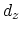orbitals of Pd and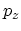orbitals of the carbon atoms, the self-energy for this SB contact can be written as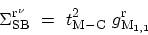(4.34)

where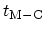is the hopping parameter between metal and carbon atoms and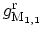is the surface GREEN's function of the metal contact. The contact model in (4.34) assumes injection from the contact into all CNT subbands.

Based on ab-initio calculations, it has been shown that the electronic band structure of the Pd-graphene system near the FERMI level can be reproduced by considering the hybridization between graphene and Pd bands, using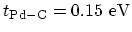.

The surface GREEN's function contains information about the band-structure of the metal contact. To calculate the surface GREEN's function, one has to specify an appropriate HAMILTONian for the contacts, for example one can employ the tight-binding method , density functional theory , or extended Hückel theory . Contacts can be approximated as semi-infinite leads along the transport axis, and infinite in the transverse direction. Therefore, the surface GREEN's function can be calculated iteratively along the transport direction (Section G.3).

Figure 4.9 shows the bulk and surface density of states of an Au-contact modeled using extended Hückel theory . The density of states and as a result the contact self-energy depend on energy. However, the density of states near the FERMI level does not vary sharply. Since transport phenomena occur mostly due to electrons close to the FERMI level, one can assume that the contact self-energy at all energies is equal to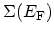. For Pd contacts we used the results of ab-initio calculations reported in .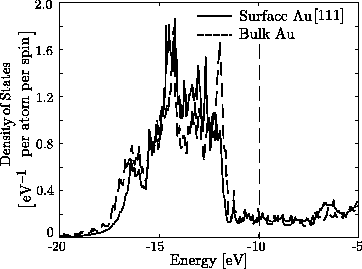M. Pourfath: Numerical Study of Quantum Transport in Carbon Nanotube-Based Transistors